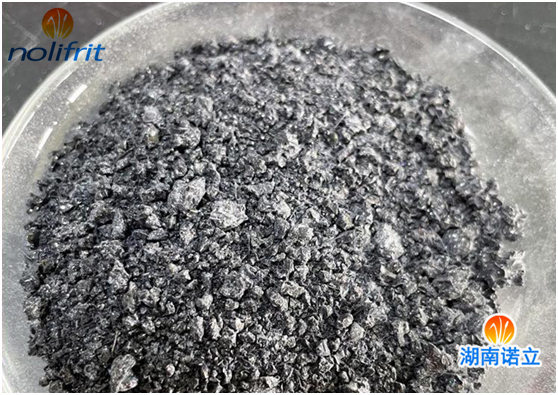# Thermal Stability of Enamel

The thermal stability of the enamel layer is the property of the enamel layer to withstand sudden temperature changes without damage. It is a comprehensive performance of properties including elasticity, thermal conductivity, specific heat capacity, thermal expansion and tensile strength. Thermal stability is related to the geometry of the enamel product. But the main thing depends on the internal structure of the enamel frit or enamel layer, that is, the number and type of crystals in the enamel layer. The enamel layer with appropriate thermal expansion coefficient, good thermal conductivity, good elasticity and high tensile strength has better thermal stability. The thermal stability of the enamel layer also has a great relationship with the thickness of the enamel layer. The thickness of the enamel layer decreases, and the thermal stability also increases.At present, there is no calculation formula for measuring the thermal stability of the enamel layer, and the calculation formula for the thermal stability of glass can only be borrowed. The thermal stability of glass can be expressed by the following formula:In the formula: K——the thermal stability of the glass;

P is the tensile strength of the glass;

E is the elastic modulus of the glass;

αl——the coefficient of linear expansion of glass;

λ——the thermal conductivity of glass;

C is the specific heat capacity of the glass;

P - the density of the glass.

The smaller the thermal stability K value, the worse the thermal stability of the enamel layer.

It can be seen from the above formula that the changes of the elastic modulus E and the tensile strength P are corresponding, so the change of the P/E value is small. In addition, the value of [λ/(cd)]1/2 is also small, so the relationship between K and the linear expansion coefficient αl is the largest, that is, the thermal stability of the glass is mainly determined by the thermal expansion coefficient of the glass, that is, K and 1 /α is proportional to.

For the enamel layer, the K value is also related to the thickness of the enamel layer. Generally speaking, K is proportional to 1/d (d is the thickness of the enamel layer).

The thermal stability of the enamel layer is also related to the mechanical strength of the green tire (excluding the thickness of the green tire material), with high mechanical strength and good thermal stability.